Open in App
Not now

# Different ways to create Pandas Dataframe

• Difficulty Level : Medium
• Last Updated : 11 Jan, 2023

Pandas DataFrame is a 2-dimensional labeled data structure like any table with rows and columns. The size and values of the dataframe are mutable,i.e., can be modified. It is the most commonly used pandas object. Pandas DataFrame can be created in multiple ways. Let’s discuss different ways to create a DataFrame one by one.

DataFrame() function is used to create a dataframe in Pandas. The syntax of creating dataframe is:

`pandas.DataFrame(data, index, columns)`

where,

data: It is a dataset from which dataframe is to be created. It can be list, dictionary, scalar value, series, ndarrays, etc.

index: It is optional, by default the index of the dataframe starts from 0 and ends at the last data value(n-1). It defines the row label explicitly.

columns: This parameter is used to provide column names in the dataframe. If the column name is not defined by default, it will take a value from 0 to n-1.

Method #0:Creating an Empty DataFrame

## Python3

 `# Importing Pandas to create DataFrame``import` `pandas as pd`` ` `# Creating Empty DataFrame and Storing it in variable df``df ``=` `pd.DataFrame()`` ` `# Printing Empty DataFrame``print``(df)`

Output:

• The DataFrame() function of pandas is used to create a dataframe.
• df variable is the name of the dataframe in our example.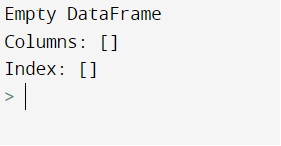Output

Method #1: Creating  Dataframe from Lists

## Python3

 `# Import pandas library``import` `pandas as pd`` ` `# initialize list elements``data ``=` `[``10``,``20``,``30``,``40``,``50``,``60``]`` ` `# Create the pandas DataFrame with column name is provided explicitly``df ``=` `pd.DataFrame(data, columns``=``[``'Numbers'``])`` ` `# print dataframe.``df`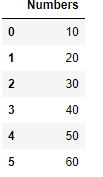Dataframe created using list

Method #2: Creating Pandas DataFrame from lists of lists.

## Python3

 `# Import pandas library``import` `pandas as pd`` ` `# initialize list of lists``data ``=` `[[``'tom'``, ``10``], [``'nick'``, ``15``], [``'juli'``, ``14``]]`` ` `# Create the pandas DataFrame``df ``=` `pd.DataFrame(data, columns``=``[``'Name'``, ``'Age'``])`` ` `# print dataframe.``df`

Output: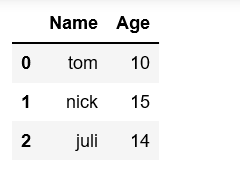Method #3: Creating DataFrame from dict of narray/lists
To create DataFrame from dict of narray/list, all the narray must be of same length. If index is passed then the length index should be equal to the length of arrays. If no index is passed, then by default, index will be range(n) where n is the array length.

## Python3

 `# Python code demonstrate creating``# DataFrame from dict narray / lists``# By default addresses.`` ` `import` `pandas as pd`` ` `# initialize data of lists.``data ``=` `{``'Name'``: [``'Tom'``, ``'nick'``, ``'krish'``, ``'jack'``],``        ``'Age'``: [``20``, ``21``, ``19``, ``18``]}`` ` `# Create DataFrame``df ``=` `pd.DataFrame(data)`` ` `# Print the output.``df`

Output: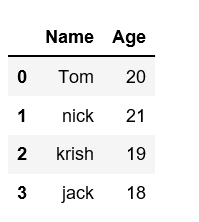Note: While creating dataframe using dictionary, the keys of dictionary will be column name by default. We can also provide column name explicitly using column parameter.

Method #4: Creating a DataFrame by proving index label explicitly.

## Python3

 `# Python code demonstrate creating``# pandas DataFrame with indexed by`` ` `# DataFrame using arrays.``import` `pandas as pd`` ` `# initialize data of lists.``data ``=` `{``'Name'``: [``'Tom'``, ``'Jack'``, ``'nick'``, ``'juli'``],``        ``'marks'``: [``99``, ``98``, ``95``, ``90``]}`` ` `# Creates pandas DataFrame.``df ``=` `pd.DataFrame(data, index``=``[``'rank1'``,``                               ``'rank2'``,``                               ``'rank3'``,``                               ``'rank4'``])`` ` `# print the data``df`

Output:Method #5: Creating Dataframe from list of dicts
Pandas DataFrame can be created by passing lists of dictionaries as a input data. By default dictionary keys will be taken as columns.

## Python3

 `# Python code demonstrate how to create``# Pandas DataFrame by lists of dicts.``import` `pandas as pd`` ` `# Initialize data to lists.``data ``=` `[{``'a'``: ``1``, ``'b'``: ``2``, ``'c'``: ``3``},``        ``{``'a'``: ``10``, ``'b'``: ``20``, ``'c'``: ``30``}]`` ` `# Creates DataFrame.``df ``=` `pd.DataFrame(data)`` ` `# Print the data``df`

Output: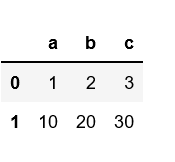Another example to create pandas DataFrame by passing lists of dictionaries and row indexes.

## Python3

 `# Python code demonstrate to create``# Pandas DataFrame by passing lists of``# Dictionaries and row indices.``import` `pandas as pd`` ` `# Initialize data of lists``data ``=` `[{``'b'``: ``2``, ``'c'``: ``3``}, {``'a'``: ``10``, ``'b'``: ``20``, ``'c'``: ``30``}]`` ` `# Creates pandas DataFrame by passing``# Lists of dictionaries and row index.``df ``=` `pd.DataFrame(data, index``=``[``'first'``, ``'second'``])`` ` `# Print the data``df`

Output: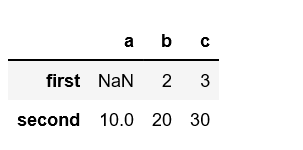Another example to create pandas DataFrame from lists of dictionaries with both row index as well as column index.

## Python3

 `# Python code demonstrate to create a``# Pandas DataFrame with lists of``# dictionaries as well as``# row and column indexes.`` ` `import` `pandas as pd`` ` `# Initialize lists data.``data ``=` `[{``'a'``: ``1``, ``'b'``: ``2``},``        ``{``'a'``: ``5``, ``'b'``: ``10``, ``'c'``: ``20``}]`` ` `# With two column indices, values same``# as dictionary keys``df1 ``=` `pd.DataFrame(data, index``=``[``'first'``,``                                ``'second'``],``                   ``columns``=``[``'a'``, ``'b'``])`` ` `# With two column indices with``# one index with other name``df2 ``=` `pd.DataFrame(data, index``=``[``'first'``,``                                ``'second'``],``                   ``columns``=``[``'a'``, ``'b1'``])`` ` `# print for first data frame``print``(df1, ``"\n"``)`` ` `# Print for second DataFrame.``print``(df2)`

Output: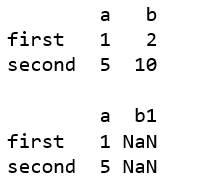Method #6: Creating DataFrame using zip() function.
Two lists can be merged by using list(zip()) function. Now, create the pandas DataFrame by calling pd.DataFrame() function.

## Python3

 `# Python program to demonstrate creating``# pandas Dataframe from lists using zip.`` ` `import` `pandas as pd`` ` `# List1``Name ``=` `[``'tom'``, ``'krish'``, ``'nick'``, ``'juli'``]`` ` `# List2``Age ``=` `[``25``, ``30``, ``26``, ``22``]`` ` `# get the list of tuples from two lists.``# and merge them by using zip().``list_of_tuples ``=` `list``(``zip``(Name, Age))`` ` `# Assign data to tuples.``list_of_tuples`` ` ` ` `# Converting lists of tuples into``# pandas Dataframe.``df ``=` `pd.DataFrame(list_of_tuples,``                  ``columns``=``[``'Name'``, ``'Age'``])`` ` `# Print data.``df`

Output: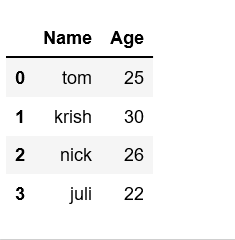Method#7:  Creating dataframe from series

To create a dataframe from series, we must pass series as argument to DataFrame() function.

## Python3

 `# Python code demonstrate creating``# Pandas Dataframe from series.`` ` `import` `pandas as pd`` ` `# Initialize data to series.``d ``=`  `pd.Series([``10``, ``20``, ``30``, ``40``])``# creates Dataframe.``df ``=` `pd.DataFrame(d)`` ` `# print the data.``df`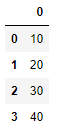Method #8: Creating DataFrame from Dictionary of series.
To create DataFrame from Dict of series, dictionary can be passed to form a DataFrame. The resultant index is the union of all the series of passed indexed.

## Python3

 `# Python code demonstrate creating``# Pandas Dataframe from Dicts of series.`` ` `import` `pandas as pd`` ` `# Initialize data to Dicts of series.``d ``=` `{``'one'``: pd.Series([``10``, ``20``, ``30``, ``40``],``                      ``index``=``[``'a'``, ``'b'``, ``'c'``, ``'d'``]),``     ``'two'``: pd.Series([``10``, ``20``, ``30``, ``40``],``                      ``index``=``[``'a'``, ``'b'``, ``'c'``, ``'d'``])}`` ` `# creates Dataframe.``df ``=` `pd.DataFrame(d)`` ` `# print the data.``df`

Output: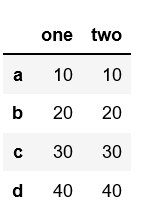My Personal Notes arrow_drop_up